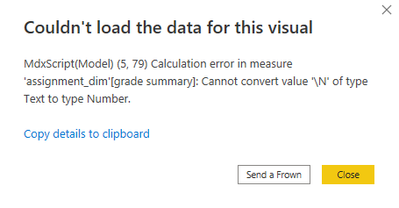cancel
Showing results for
Did you mean:Helper I

## Summary of results from a table

We have a cybersecurity course that we require all student employees to take when they are hired. Their managers are supposed to verify they have completed this, but usually it is a low priority for them. We'd like to give the managers a reminder if a student does not complete the course or fails it.

The students must complete all of the assessments and pass the course with an 80%. The exam they take is weighted double of each assignment. There is also a pre-course test they take to measure their current knowledge of the subject. The pre-course test is required, but not calculated into the final grade. If they get less than 80%, they need to retake the course.

The table I have shows their results like this:

name, assessment, score

employee1, pretest, 80

employee1, assignment1, 80

employee1, assignment2, 80

employee1, exam, 160

employee2, pretest, 100

employee2, assignment1, 80

employee2, assignment2, 80

employee3, pretest, 50

employee3, assignment1, 80

employee3, assignment2, 80

employee3, exam, 140

In the example above, employee1 has an 80 on all assessments and has passed the course. employee2 has an 80% on 3 of the assessments, but did not take the exam. employee3 completed all assessments, but failed to get an 80%.

What I'd like to get is something like this:

name, score, status

employee1, 80%, passed

employee2, 80%, incomplete

employee3, 75%, failed

I know the formula for calculating the final grade is simply (assignment1+assignment2+exam)/400, and the pass/fail would be final grade >= 80 or final grade < 80. Not sure how to get started on the summarized results though. Any guidance would be appreciated!

4 REPLIES 4Resolver I

Hi,

I would start with using the advanced editor in power query to set out the conditions for passing where PREVIOUSSTEP is your previous step:

``````    #"Added Custom" = Table.AddColumn(#"Renamed Columns", "Pretest taken", each if [assessment] = "pretest" and [score] <> null then "Y" else "N"),
#"Added Custom1" = Table.AddColumn(#"Added Custom", "Assignment passed", each if [assessment] = "assignment1" and [score] >= 80 then "Y" else "N"),
#"Added Custom2" = Table.AddColumn(PREVIOUSSTEP, "Exam taken", each if [assessment] = "exam" then "Y" else "N"),
#"Grouped Rows" = Table.Group(#"Added Custom2", {"name"}, {{"Pretest", each List.Max([Pretest taken]), type text}, {"Assignment", each List.Max([Assignment passed]), type text}, {"Exam", each List.Max([Exam taken]), type text}, {"Score", each _, type table [name=nullable text, assessment=nullable text, score=nullable number, Pretest taken=text, Assignment passed=text, Exam taken=text]}}),
#"Expanded Score" = Table.ExpandTableColumn(#"Grouped Rows", "Score", {"assessment", "score"}, {"assessment", "score.1"}),
#"Filtered Rows1" = Table.SelectRows(#"Expanded Score", each ([assessment] <> "pretest"))
in
#"Filtered Rows1"``````

Next, I would create a column in DAX to calculate the final score and change the type to percentage afterwards.

``````Score =
var overallscore = CALCULATE( sum ('Table'[score.1]), FILTER('Table', [name] = EARLIER([name])))
return
divide (overallscore,400, blank())``````

then, I would create another DAX column to find the state:

``State = if([Score] >= 0.8 && [Exam] = "Y", "Passed", "Failed")``

Not sure yet how to do "incomplete" status! but this is halfway there:

``incomplete = if ( [Exam] = "N", SWITCH([State], "Failed", "Incomplete"))``Super User

@power-bi-noob , Try a measure like

Divide( countrows(filter(Table, Table[score] > 80)),countrows(Table))Helper I

Hi @amitchandak , I think that is taking me closer.

First run gets me this error:The grades are stored as text, not integers.

Then I tried this.

and got this error:I modified it further to try and use a conditional , but then the last argument shows up as an an unexpected value.

,countrows(submission_dim))

Not sure where I'm going wrong here, all of my parenthesis appear to be balanced.Super User

@power-bi-noob , oh Grade is text ?

Try like